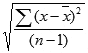The STDEV.S (STDEV S) is a Statistical function that calculates and returns the standard deviation for a sample of data. The standard deviation is a measure of how widely values are dispersed from the average value (the mean). The STDEV.S calculates standard deviation using the "n-1" method. In this guide, we’re going to show you how to use the STDEV.S function and also go over some tips and error handling methods.

## Supported versions

• Excel 2010 and later

## Syntax

STDEV.S(number1,[number2],...)

## Arguments

 number1 The first number or reference in the sample of the population. You can also use an array instead of multiple arguments. [number2], … Other numbers of the population up to 254 arguments.

## Example

The standard deviation is a measure of how widely values are dispersed from the average value (the mean). The STDEV.S function assumes that given arguments are a sample of the population. The function uses the following formula:where x is the average (mean) and n is the sample size.

All you need to do is to supply your dataset as arguments.

=STDEV.S(B5:B13)## Tips

• Use STDEV.S to calculate the standard deviation for a sample of the population.
• The function calculates the standard deviation by using the "n-1" method.
• The STDEV.P function uses "n" method to calculate the standard deviation.
• You can use the AVERAGE function to calculate the mean of a data set.
• The STDEV is the older version of this function. Refrain from using STDEV.
• Arguments can either be numbers or names, arrays, or references that contain numbers.
• Logical values, and text representations of numbers that you type directly into the list of arguments are counted.
• If an argument is an array or reference, only numbers in that array or reference are counted. Empty cells, logical values, text, or error values in the array or reference are ignored.
• Arguments that are error values or text that cannot be translated into numbers cause errors.

If you want to include logical values and text representations of numbers in a reference as part of the calculation, use the STDEVA function.